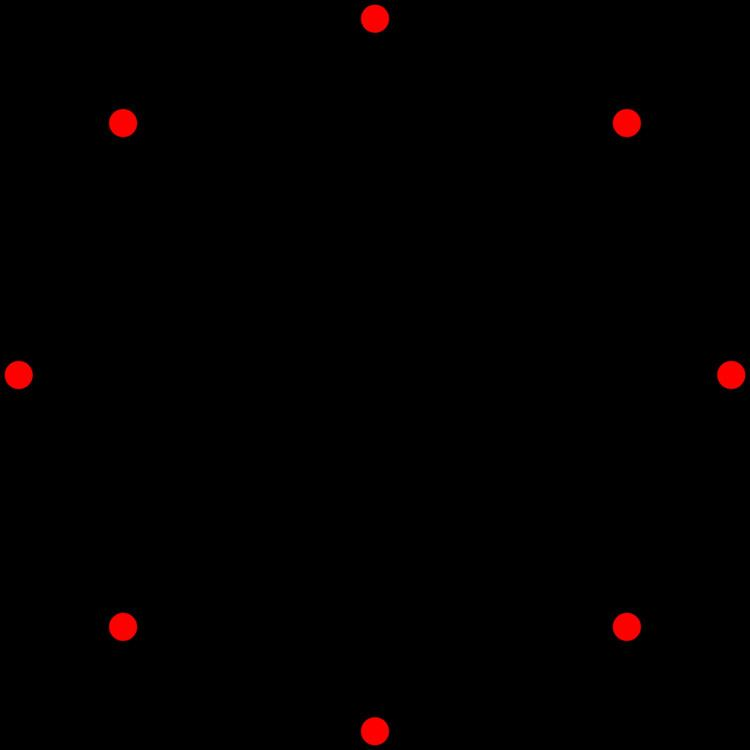# A7 polytope

Updated onIn 7-dimensional geometry, there are 71 uniform polytopes with A7 symmetry. There is one self-dual regular form, the 7-simplex with 8 vertices.

Each can be visualized as symmetric orthographic projections in Coxeter planes of the A7 Coxeter group, and other subgroups.

## Graphs

Symmetric orthographic projections of these 135 polytopes can be made in the A7, A6, A5, A4, A3, A2 Coxeter planes. Ak has [k+1] symmetry. For even k and symmetrically ringed-diagrams, symmetry doubles to [2(k+1)].

These 63 polytopes are each shown in these 6 symmetry planes, with vertices and edges drawn, and vertices colored by the number of overlapping vertices in each projective position.

A7 polytope Wikipedia